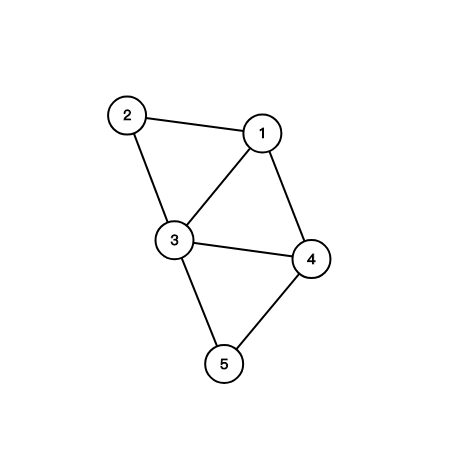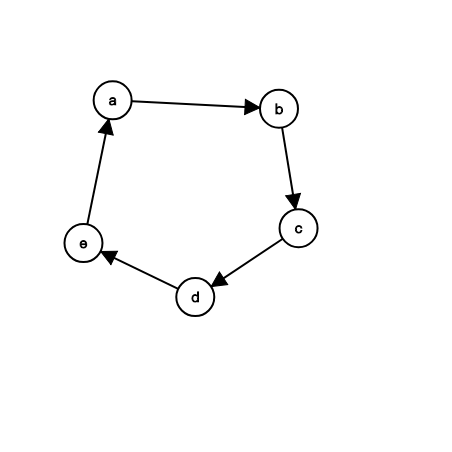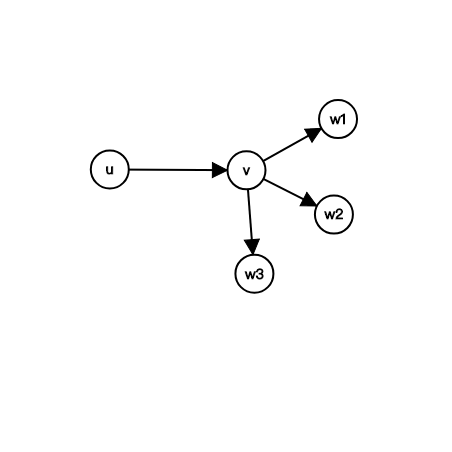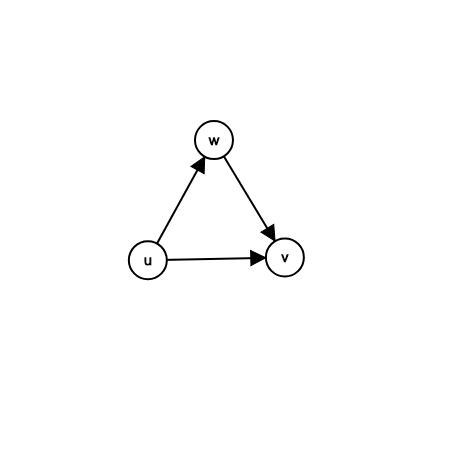# 三元环计数

1. 有一条连接$i,j$的边
2. 有一条连接$j,k$的边
3. 有一条连接$k,i$的边1. 定向后的图是一个有向无环图1. 时间复杂度是$O(m \sqrt m)$（在此认为$n,m$同阶）1. $out_v \le \sqrt m$，那么由于$u$连接$v$，因此$deg_u \ge deg_v$，这样的$u$的个数是$O(n)$的，因此这里的时间复杂度为$O(n \sqrt m)$
2. $out_v \gt \sqrt m$，那么由于$u$连接$v$，因此$deg_u \ge deg_v$，既$deg_u \gt \sqrt m$，这样的$u$的个数是$O(\sqrt m)$的，因此这里的时间复杂度为$O(\sqrt m m)=O(m \sqrt m)$

1. 每个三元环只会被统计一次• 微信扫一扫